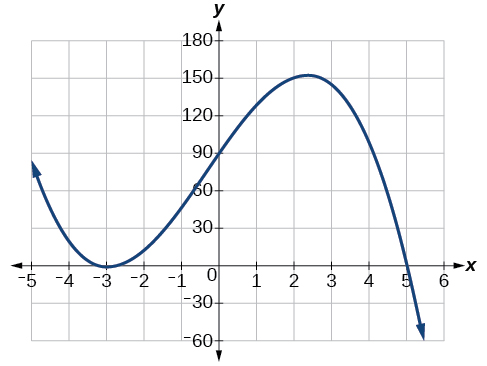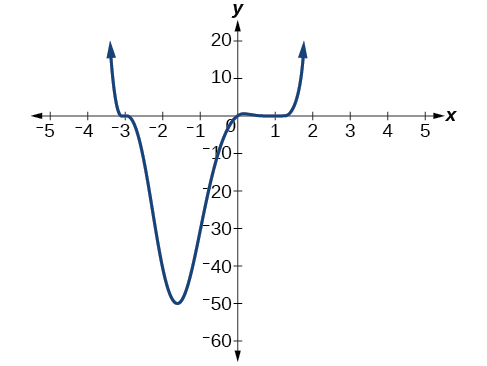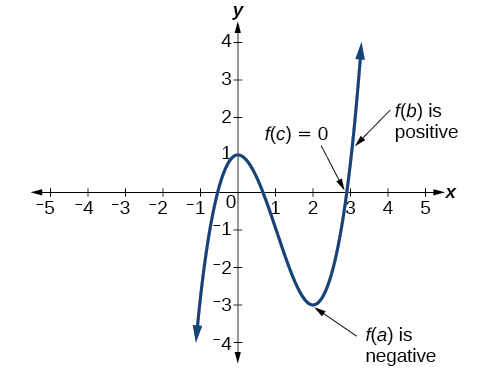# 5.3 Graphs of polynomial functions  (Page 5/13)

 Page 5 / 13

## Sketching the graph of a polynomial function

Sketch a graph of $\text{\hspace{0.17em}}f\left(x\right)=-2{\left(x+3\right)}^{2}\left(x-5\right).$

This graph has two x -intercepts. At $\text{\hspace{0.17em}}x=-3,\text{\hspace{0.17em}}$ the factor is squared, indicating a multiplicity of 2. The graph will bounce at this x -intercept. At $\text{\hspace{0.17em}}x=5,\text{\hspace{0.17em}}$ the function has a multiplicity of one, indicating the graph will cross through the axis at this intercept.

The y -intercept is found by evaluating $\text{\hspace{0.17em}}f\left(0\right).$

$\begin{array}{ccc}\hfill f\left(0\right)& =& -2{\left(0+3\right)}^{2}\left(0-5\right)\hfill \\ & =& -2\cdot 9\cdot \left(-5\right)\hfill \\ & =& 90\hfill \end{array}$

The y -intercept is $\text{\hspace{0.17em}}\left(0,90\right).$

Additionally, we can see the leading term, if this polynomial were multiplied out, would be $\text{\hspace{0.17em}}-2{x}^{3},\text{\hspace{0.17em}}$ so the end behavior is that of a vertically reflected cubic, with the outputs decreasing as the inputs approach infinity, and the outputs increasing as the inputs approach negative infinity. See [link] .

To sketch this, we consider that:

• As $\text{\hspace{0.17em}}x\to -\infty \text{\hspace{0.17em}}$ the function $\text{\hspace{0.17em}}f\left(x\right)\to \infty ,\text{\hspace{0.17em}}$ so we know the graph starts in the second quadrant and is decreasing toward the $\text{\hspace{0.17em}}x\text{-}$ axis.
• Since $\text{\hspace{0.17em}}f\left(-x\right)=-2{\left(-x+3\right)}^{2}\left(-x–5\right)\text{\hspace{0.17em}}$ is not equal to $\text{\hspace{0.17em}}f\left(x\right),\text{\hspace{0.17em}}$ the graph does not display symmetry.
• At $\text{\hspace{0.17em}}\left(-3,0\right),\text{\hspace{0.17em}}$ the graph bounces off of the x -axis, so the function must start increasing.

At $\text{\hspace{0.17em}}\left(0,90\right),\text{\hspace{0.17em}}$ the graph crosses the y -axis at the y -intercept. See [link] .

Somewhere after this point, the graph must turn back down or start decreasing toward the horizontal axis because the graph passes through the next intercept at $\text{\hspace{0.17em}}\left(5,0\right).\text{\hspace{0.17em}}$ See [link] .

As $\text{\hspace{0.17em}}x\to \infty \text{\hspace{0.17em}}$ the function $\text{\hspace{0.17em}}f\left(x\right)\to \mathrm{-\infty },\text{\hspace{0.17em}}$ so we know the graph continues to decrease, and we can stop drawing the graph in the fourth quadrant.

Using technology, we can create the graph for the polynomial function, shown in [link] , and verify that the resulting graph looks like our sketch in [link] .The complete graph of the polynomial function   f ( x ) = − 2 ( x + 3 ) 2 ( x − 5 )

Sketch a graph of $\text{\hspace{0.17em}}f\left(x\right)=\frac{1}{4}x{\left(x-1\right)}^{4}{\left(x+3\right)}^{3}.$## Using the intermediate value theorem

In some situations, we may know two points on a graph but not the zeros. If those two points are on opposite sides of the x -axis, we can confirm that there is a zero between them. Consider a polynomial function $\text{\hspace{0.17em}}f\text{\hspace{0.17em}}$ whose graph is smooth and continuous. The Intermediate Value Theorem    states that for two numbers $\text{\hspace{0.17em}}a\text{\hspace{0.17em}}$ and $\text{\hspace{0.17em}}b\text{\hspace{0.17em}}$ in the domain of $\text{\hspace{0.17em}}f,$ if $\text{\hspace{0.17em}}a and $f\left(a\right)\ne f\left(b\right),$ then the function $\text{\hspace{0.17em}}f\text{\hspace{0.17em}}$ takes on every value between $\text{\hspace{0.17em}}f\left(a\right)\text{\hspace{0.17em}}$ and $\text{\hspace{0.17em}}f\left(b\right).\text{\hspace{0.17em}}$ (While the theorem is intuitive, the proof is actually quite complicated and requires higher mathematics.) We can apply this theorem to a special case that is useful in graphing polynomial functions. If a point on the graph of a continuous function $\text{\hspace{0.17em}}f\text{\hspace{0.17em}}$ at $\text{\hspace{0.17em}}x=a\text{\hspace{0.17em}}$ lies above the $\text{\hspace{0.17em}}x\text{-}$ axis and another point at $\text{\hspace{0.17em}}x=b\text{\hspace{0.17em}}$ lies below the $\text{\hspace{0.17em}}x\text{-}$ axis, there must exist a third point between $\text{\hspace{0.17em}}x=a\text{\hspace{0.17em}}$ and $\text{\hspace{0.17em}}x=b\text{\hspace{0.17em}}$ where the graph crosses the $\text{\hspace{0.17em}}x\text{-}$ axis. Call this point This means that we are assured there is a solution $\text{\hspace{0.17em}}c\text{\hspace{0.17em}}$ where $f\left(c\right)=0.$

In other words, the Intermediate Value Theorem tells us that when a polynomial function changes from a negative value to a positive value, the function must cross the $\text{\hspace{0.17em}}x\text{-}$ axis. [link] shows that there is a zero between $\text{\hspace{0.17em}}a\text{\hspace{0.17em}}$ and $\text{\hspace{0.17em}}b.\text{\hspace{0.17em}}$Using the Intermediate Value Theorem to show there exists a zero.

## Intermediate value theorem

Let $\text{\hspace{0.17em}}f\text{\hspace{0.17em}}$ be a polynomial function. The Intermediate Value Theorem    states that if $\text{\hspace{0.17em}}f\left(a\right)\text{\hspace{0.17em}}$ and $\text{\hspace{0.17em}}f\left(b\right)\text{\hspace{0.17em}}$ have opposite signs, then there exists at least one value $\text{\hspace{0.17em}}c\text{\hspace{0.17em}}$ between $\text{\hspace{0.17em}}a\text{\hspace{0.17em}}$ and $\text{\hspace{0.17em}}b\text{\hspace{0.17em}}$ for which $\text{\hspace{0.17em}}f\left(c\right)=0.$

#### Questions & Answers

x exposant 4 + 4 x exposant 3 + 8 exposant 2 + 4 x + 1 = 0
x exposent4+4x exposent3+8x exposent2+4x+1=0
HERVE
How can I solve for a domain and a codomains in a given function?
ranges
EDWIN
Thank you I mean range sir.
Oliver
proof for set theory
don't you know?
Inkoom
find to nearest one decimal place of centimeter the length of an arc of circle of radius length 12.5cm and subtending of centeral angle 1.6rad
factoring polynomial
find general solution of the Tanx=-1/root3,secx=2/root3
find general solution of the following equation
Nani
the value of 2 sin square 60 Cos 60
0.75
Lynne
0.75
Inkoom
when can I use sin, cos tan in a giving question
depending on the question
Nicholas
I am a carpenter and I have to cut and assemble a conventional roof line for a new home. The dimensions are: width 30'6" length 40'6". I want a 6 and 12 pitch. The roof is a full hip construction. Give me the L,W and height of rafters for the hip, hip jacks also the length of common jacks.
John
I want to learn the calculations
where can I get indices
I need matrices
Nasasira
hi
Raihany
Hi
Solomon
need help
Raihany
maybe provide us videos
Nasasira
Raihany
Hello
Cromwell
a
Amie
What do you mean by a
Cromwell
nothing. I accidentally press it
Amie
you guys know any app with matrices?
Khay
Ok
Cromwell
Solve the x? x=18+(24-3)=72
x-39=72 x=111
Suraj
Solve the formula for the indicated variable P=b+4a+2c, for b
Need help with this question please
b=-4ac-2c+P
Denisse
b=p-4a-2c
Suddhen
b= p - 4a - 2c
Snr
p=2(2a+C)+b
Suraj
b=p-2(2a+c)
Tapiwa
P=4a+b+2C
COLEMAN
b=P-4a-2c
COLEMAN
like Deadra, show me the step by step order of operation to alive for b
John
A laser rangefinder is locked on a comet approaching Earth. The distance g(x), in kilometers, of the comet after x days, for x in the interval 0 to 30 days, is given by g(x)=250,000csc(π30x). Graph g(x) on the interval [0, 35]. Evaluate g(5)  and interpret the information. What is the minimum distance between the comet and Earth? When does this occur? To which constant in the equation does this correspond? Find and discuss the meaning of any vertical asymptotes.
The sequence is {1,-1,1-1.....} hasByByByByBy David Martin